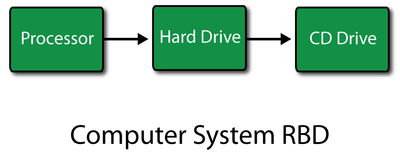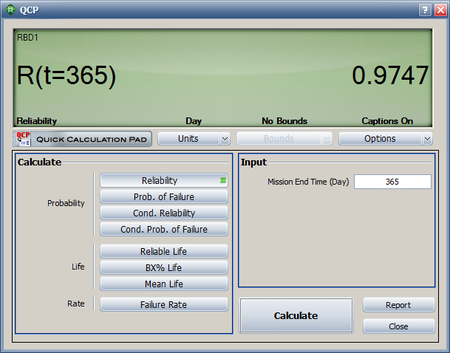# Example Calculating System Reliability with Duty Cycles

This example appears in the System Analysis Reference book.

Consider a computer system with three components: a processor, a hard drive and a CD drive in series as shown next. Assume that all three components follow a Weibull failure distribution. The processor has the following parameters, ${{\beta }_{1}}=1.5\,\!$ and ${{\eta }_{1}}=5000\,\!$. For the hard drive, the parameters are ${{\beta }_{2}}=2.5\,\!$ and ${{\eta }_{2}}=3000\,\!$, and for the CD drive they are ${{\beta }_{3}}=2\,\!$ and ${{\eta }_{3}}=4000\,\!$. Determine the reliability of the computer system after one year (365 days) of operation, assuming that the CD drive is used only 30% of the time.Solution

The reliability of the processor after 365 days of operation is given by:

\begin{align} {{R}_{processor}}(365)= & {{e}^{-{{\left( \tfrac{365}{{{\eta }_{1}}} \right)}^{{{\beta }_{1}}}}}} \\ = & {{e}^{-{{\left( \tfrac{365}{5000} \right)}^{1.5}}}} \\ = & 0.9805\text{ or }98.05% \end{align}\,\!

The reliability of the hard drive after 365 days of operation is given by:

\begin{align} {{R}_{harddrive}}(365)= & {{e}^{-{{\left( \tfrac{365}{{{\eta }_{2}}} \right)}^{{{\beta }_{2}}}}}} \\ = & {{e}^{-{{\left( \tfrac{365}{3000} \right)}^{2.5}}}} \\ = & 0.9948\text{ or }99.48% \end{align}\,\!

The reliability of the CD drive after 365 days of operation (taking into account the 30% operation using a duty cycle of 0.3) is given by:

\begin{align} {{R}_{CDdrive}}(365)= & {{e}^{-{{\left( \tfrac{{{d}_{c}}\times 365}{{{\eta }_{3}}} \right)}^{{{\beta }_{3}}}}}} \\ = & {{e}^{-{{\left( \tfrac{0.3\times 365}{4000} \right)}^{2}}}} \\ = & 0.9993\text{ or }99.93% \end{align}\,\!

Thus the reliability of the computer system after 365 days of operation is:

\begin{align} {{R}_{s}}(365)= & {{R}_{processor}}(365)\cdot {{R}_{harddrive}}(365)\cdot {{R}_{CDdrive}}(365) \\ = & 0.9805\cdot 0.9948\cdot 0.9993 \\ = & 0.9747\text{ or }97.47% \end{align}\,\!

The result can also be obtained in BlockSim by creating an RBD of the system and using the Quick Calculation Pad (QCP) to calculate the reliability, as shown in the following figure.